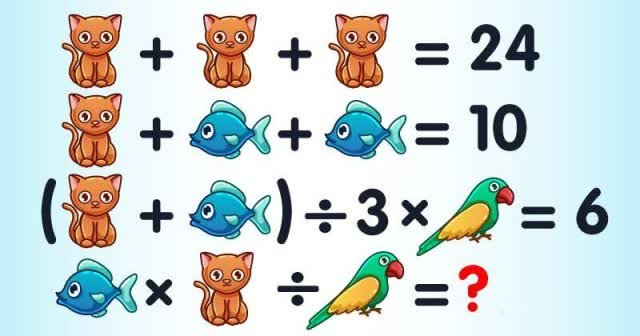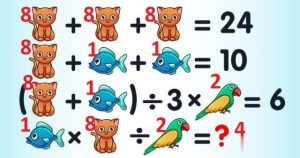# This Math Puzzle Is Causing A Stir On The Internet – But Can You Solve It?

On the internet, a math riddle has been generating a sensation when viewers were perplexed by the solution.

It’s time to recall all you learnt in school and give your brain a boost by participating in an arithmetic puzzle that most people find difficult to complete.

Let’s speak about some ground rules before we get started. Four equations may be seen in the image above. Your task is to use the first three lines to determine the answer to the fourth equation.

Remember that the three icons, which include a parrot, a fish, and a cat, all have distinct values, and there is only one correct answer.

Now it’s time to roll up your sleeves and get to work! Oh, and don’t forget about PEMDAS, too.

In every current order of operations, multiplication and division are always given the same precedence. As a result, you should always begin on the left side of the equation and work your way to the right.

Scroll down to see the solution after you’re confident you’ve got the right answer.

The answer is 4! Here’s how we got to that number.

First of all, let’s assume that C stands for cat, F for fish, and P for parrot.

LINE 1: C + C + C = 24 and 3C = 24. Therefore, C = 8.

LINE 2: C + F + F = 10 and 8 + 2F = 10. Therefore, 2F = 2 and F = 1.

LINE 3: (C + F) ÷ 3 × P = 6 and (9) ÷ 3 × P = 6. Therefore, 3 × P = 6 and P = 2.

LINE 4: F × C ÷ P = 1 × 8 ÷ 2 = 8 ÷ 2 = 4